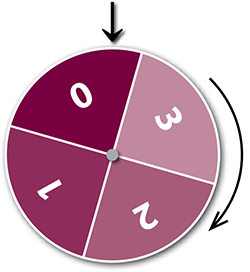The Student Puzzle Corner contains problems in statistics or probability. Solving them may require a literature search.
Student IMS members are invited to submit solutions (to bulletin@imstat.org with subject “Student Puzzle Corner”). The deadline is January 15, 2018. The names and affiliations of student members who submit correct solutions, and the answer, will be published in the following issue. The Puzzle Editor’s decision is final.

### Guest contributor Professor Stanislav Volkov, Centre for Mathematical Sciences at Lund University in Sweden, poses this issue’s puzzle:Suppose that you have a rotating wheel with $M=4$ numbers “0″,“1″,“2″,“3″ equidistantly written on it, and a sequence of probabilities $p_1,p_2,\dots$. At time $n=0$ the wheel has “0″ on the top.

Let $Y_n \in\{0,1,2,3\}$ be the number which is shown on the top of the wheel at time $n$. For each time $n\ge 1$, with probability $p_n$, independently of the past, you rotate the wheel $90$ degrees clockwise, so that $Y_{n+1}$ becomes $(Y_n+1) \mod 4$. With the remaining probability you do nothing.

Now assume also that $p_n$ is summable; then by the Borel-Cantelli Lemma you rotate the wheel only finitely many times; consequently $Y_n=Y_\infty$ for all sufficiently large~$n$.

Question: given $Y_0=0$, what is probability that $Y_\infty=0$?

The answer should be quite a simple formula as a function of $p_n$. In particular, if $p_n=\frac 1{2n^2+1}$, show that this probability is just barely smaller than $1/2$, namely
$$\frac 14+\frac{\sqrt 2 \cosh(\pi/2)+\sin(\pi/\sqrt 2)} {4 \sinh (\pi/\sqrt 2)}.$$

### Contributing Editor Anirban DasGupta, on the solution to the previous puzzleColman Humphrey, a PhD student at the Wharton School, University of Pennsylvania, sent a solution.

In general, this is a very hard problem. The likelihood function is a finite mixture of Gauss’s hypergeometric functions and it may have multiple local maxima. However, it is bounded and continuous, and has a maximum. The minimax solution for fixed sample sizes would be nearly impossible, or actually impossible to find. I do not know what a minimax solution is. If we make the parameter space compact, there is a Bayes minimax solution. It is not Bayes against a Beta prior. However, one cannot do much better than the obvious natural estimate, whether it is truncated or not. As the respondent points out, consistency and asymptotically correct confidence intervals are trivially achieved. The problem was first proposed in Rao, 1952, Advanced Statistical Methods in Biometric Research.